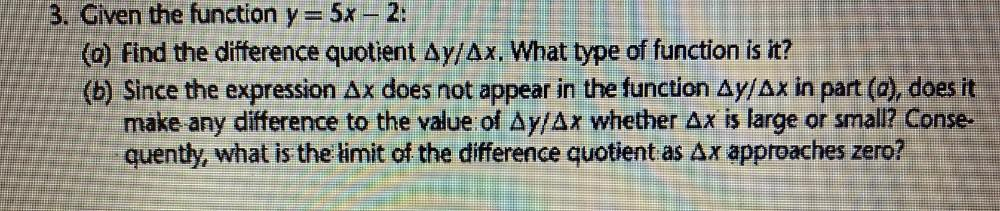Question:

# 3. Given the function y = 5x – 2: (a) Find the difference quotient Ay/Ax. What type of function is it? (b) Since the expression3. Given the function y = 5x – 2: (a) Find the difference quotient Ay/Ax. What type of function is it? (b) Since the expression Ax does not appear in the function Ay/Ax in part (a), does it make any difference to the value of Ay/Ax whether Ax is large or small? Conse quently, what is the limit of the difference quotient as Ax approaches zero?